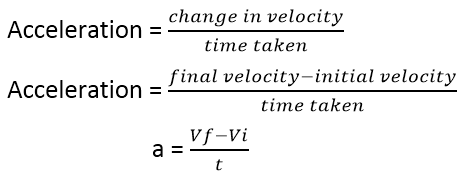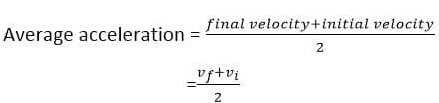Mechanics

# Acceleration formula with types

## Acceleration formula

This is a complete guide to learn how to find acceleration in Physics by using an acceleration formula.
So if you want to get benefits from this guide, you’ll enjoy the actionable tips in this new guide.
This guide includes:

• Acceleration definition
• Acceleration formula
• Real-life examples
• Types
• More

Let’s dive right in.

## What is acceleration?

In Physics, Acceleration is defined as the rate of change of velocity of a body with respect to time. When the velocity of a body changes it is said to be accelerating. Accelerations are the vector quantities. The meter per second square ( m/s²) is the acceleration unit. Positive acceleration and negative acceleration are two types of acceleration.

### What is Acceleration formula?

We can find acceleration by the following formula and from Newton’s second law of motion.Taking an as an acceleration, initial velocity as Vi, final velocity as Vf and t as the time interval, the SI unit of acceleration is meter per second per second ms-2

### Acceleration in relation to force

On the other hand, Newtonian mechanics establishes for a body of constant mass (m), contemplated by an inertial observer, a relationship of proportionality with respect to the force applied to the object (F) and the acceleration obtained (a), that is:
F = m. to
This relationship is valid in any inertial reference system and allows the acceleration to be calculated with the following formula:
a = F / m
This formulation obeys Newton’s Second Law.

### Acceleration Examples

Some examples of acceleration are given in the list below:
1.- When you press the accelerator pedal in a car, the car moves faster and faster. This change in speed is acceleration.
2.- If the same force is used to push a truck and push a car, the car will have more acceleration than the truck, because the car has less mass.
3.- It is easier to push an empty shopping cart than a full one, because the full shopping cart has more mass than the empty one.
4.- An object was moving north at 10 meters per second. The object accelerates and is now moving north at 15 meters per second. The object has been accelerated.
5.- When propelling the skateboard with our foot, there is a change in acceleration. Let’s go faster.

### What is average acceleration in physics?

The average acceleration of a body given in the above equation is a during time t. Let the time t is divided into many smaller intervals of time. If the rate of change of velocity during all these intervals remains constant then the acceleration also remains constant. Such a body is said to possess uniform acceleration.

#### average acceleration formula## Uniform acceleration in physics

“A body has uniform acceleration if it has equal changes in velocity in equal intervals of time however short the interval may be.”

### Types of acceleration

There are two types of acceleration:

1. Positive acceleration
2. Negative acceleration

#### What is positive acceleration?

Acceleration of a body is positive if its velocity increases with time.The direction of this acceleration is the same in which the body is moving without a change in its direction.

## Examples of positive acceleration

• When the train leaves the station its velocity increases with increasing time, this is the example of positive acceleration.
• When we start a vehicle its velocity increases with time, this is positive acceleration.

## What is negative acceleration?

Acceleration of a body is negative if the velocity of the body decreases.The direction of negative acceleration is opposite to the direction in which the body is moving. Negative acceleration is also called deceleration or retardation.

#### Examples of negative acceleration

• When a train reaches a station its velocity decreases with time, this type of acceleration is called negative acceleration.

similarly:
In example, an elevator moving upward (which we take to be the direction of positive velocity ) cane accelerate upward with positive acceleration and move faster or accelerate downward with negative acceleration and move slower.
When it is moving downward with negative velocity, it can accelerate downward with negative acceleration and move slower. When the acceleration and velocity have positive signs, so that the speed (the magnitude of the velocity) is decreasing, we refer to a deceleration.
How do you calculate the acceleration due to gravity?
“The acceleration of freely falling bodies is called gravitational acceleration.”Drop an object from some height and observe its motion.Does its velocity increase,decrease or remain constant as it approaches the ground? Galileo was the first scientist to notice that all the freely falling objects have the same acceleration independent of their masses.

### Acceleration calculation examples

1. A racing car increases its speed at a constant rate of 18.5 m / s at 46.1 m / s in 2.47 seconds. What will its average acceleration be?

a = dv / dt = (v f – v i ) / (t f – t i ) where v f = 46.1 m / s, v i = 18.5 m / s, t f = 2.47 s , t i = 0 s.
So: a = (46.1 – 18.5) / 2.47 = 11.17 m / s 2

1. A motorcyclist travels at 22.4 m / s and realizes that he has mistaken the route. Apply the brakes and the motorcycle stops in 2.55 s. What will be its slowdown?

a = dv / dt = (v f – v i ) / (t f – t i ), where v f = 0 m / s, v i = 22.4 m / s, t f = 2.55 s, t i = 0 s.
So:  a = (0 – 22.4) / 2.55 = -8.78 m / s 2

1. A force of 10 newtons of magnitude acts uniformly on a mass of 2 kilograms. What will be the acceleration of the pushed object?

a = F / m, where F = 10 N, m = 2Kg.
Thus:
a = 10/2 = 5 m / s 2

1. Someone is pulling a 400 kg piece of furniture to one side with a net force of 150 newtons. Another person pushes it in the same direction with a force of 200 newtons, but there is a wind blowing in the opposite direction with a force of 10 newtons. What will be the acceleration of the furniture?

We know that a = F / m, where the net force will be the sum of those in the same sense minus the one that opposes them: F = 150 N (person 1) + 200 N (person 2) – 10N (wind), which results in 340 N. We also know that m = 400 kg.
Then:  a = 340 N / 400 kg = 0.85 m / s 2

1. A remote-controlled aircraft, with a mass of 10 kg, flies with an acceleration of 2 m / s 2 heading North. Just then a wind blows to the east, with a force of 100 N. What will be the new acceleration of the airplane that maintains its course?

Since the force of the wind is perpendicular to the direction of movement of the airplane, it will not have any effect on its movement. It will continue accelerating north at 2 m / s 2 .

1. Two boys, one weak and one strong play tug-of-war, each at one end of the rope. The first pull to the left does so with a force of 5 N and the second pulls in the opposite direction does so with a force of 7 N. Taking into account that 1 newton (N) is equal to 1 kilogram-meter/second squared ( kg-m / s2), what will be the acceleration reached by the body of the weakest child when being dragged in the opposite direction by the other?

From F = ma we know that a = F / m, but we must find the net force, which will be 2 N (7 N for the strong child – 5 N for the weak child).
Then, we must find the mass, which for the purposes of calculation must be derived from the force that the weak child opposes, namely: 1 N = 1kg.m / s 2 , that is, it is the amount of force to mobilize a kilogram of mass at one meter per second squared.
Therefore, 5N = 5kg.m / s 2 . Hence m = 5Kg.
And finally, we know that a = 2N (F) / 5kg (m) = 0.4 m / s 2

1. A fire truck increases its speed from 0 to 21m / s to the East, in 3.5 seconds. What is its acceleration?

We know that: V i = 0 m / s, V f = 21 m / s, t = 3.5 seconds. Therefore we apply the formula:
a = dv / dt = (v f – v i ) / (t f – t i ), that is, a = 21m / s / 3.5 s = 6 m / s 2

1. A car reduces its speed from 21m / s East to 7m / s East in 3.5.0 seconds. What is its acceleration?

Knowing that V i = 21 m / s, V f = 7 m / s, t = 3.5 seconds, and that a = dv / dt = (v f – v i ) / (t f – t i ), is simple calculate it:
a = 7m / s – 21m / s / 3.5s = -4m / s 2 , that is, a negative acceleration (deceleration).

Related Topics:

External sources

• http://formulas.tutorvista.com/physics/acceleration-formula
• http://www.physicsclassroom.com/class/1DKin/Lesson-1/Acceleration